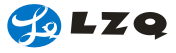Indexable (profile ) cutting tool (grinding & non-grinding) （Super high-wear-resistant/high-impact-resistanapplication）

 Tool clearance 6°, grade M, single-side chip breaker groove RCMX (RPMS) (RDMX) Insert tool(40°-60°)with countersunk & cylindrical
For example
φD=20，φd＝6.5，S＝6.35（RCMX2006MO)
φD=12，φd＝4.2，S＝4.76（RCMX1204MO)
 A

For example
φD=12，φd＝4.6，T＝5.56
 B

KPEB-R

For example
L=25.82,B=16.48,S=6.35,R=30,φd=5.5
 C
Throw away ball milling

For example
φD=8/10/12，φd＝3/4，S＝3，H=6

 D

Throw away ball (circular) milling

For example
φD=16/20/25/32，
φd＝5/6，S＝4/5/6，H=6/8

 E

Throw away ball (circular) rough milling
(profile milling)

For example
φD=20/25/32，φd＝5/6，S＝3/4/5,H=6/9

 F
T-X

For example
φD=11.2，φd＝4.4，S＝4

 G
YCF/YCE

For example
L=25.4/19.05，B=12.7/14.28,
S=6.35,R=1.5/2.0/3.0/4.0,φd＝5.4

 H
CDE-R/L

For example
L=22.8,B=14.15,S＝6.35,φd＝5.4

 I
CNE-R/L

For example
L=15.875,B=12.7,S=7.94/6.35,φd=5.5

 J
DNE-X

For example
L=12.70,B=11.12,S=6.35,φd＝4.60

 K
YCE-01

For example
L=19.05,B=14.28,S=6.35,φd＝5.4

 L
DNE-04

For example
L=12.7,B=11.12,S=6.35,φd=4.6

 M

CNE-X

For example
L=15.875,B=12.7,S=6.35/7.94,φd＝5.5

 N

LSE-R/L

For example
L=28.6/19.05,B=14.28,
S=9.525/6.35,φd＝5.4

 O
 ★Confirm machined materials, HRC, equipment rigidity (value), quantity, we can grind profile angle and dimensional tolerance according to drawings, samples, and requirements… only produce in batch, can completely replace international first-class products, having higher performance/price ratio.-193-